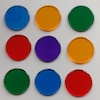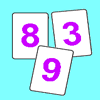# Resources tagged with: Prime numbers

Filter by: Content type:
Age range:
Challenge level:

There are 25 NRICH Mathematical resources connected to Prime numbers, you may find related items under Properties of Numbers.

Broad Topics > Properties of Numbers > Prime numbers### Factors and Multiples Game

##### Age 7 to 16Challenge Level

A game in which players take it in turns to choose a number. Can you block your opponent?### Factors and Multiples Puzzle

##### Age 11 to 14Challenge Level

Using your knowledge of the properties of numbers, can you fill all the squares on the board?### Stars

##### Age 11 to 14Challenge Level

Can you work out what step size to take to ensure you visit all the dots on the circle?### Two Primes Make One Square

##### Age 7 to 11Challenge Level

Can you make square numbers by adding two prime numbers together?### Factor Lines

##### Age 7 to 14Challenge Level

Arrange the four number cards on the grid, according to the rules, to make a diagonal, vertical or horizontal line.### One to Eight

##### Age 11 to 14Challenge Level

Complete the following expressions so that each one gives a four digit number as the product of two two digit numbers and uses the digits 1 to 8 once and only once.### Round and Round the Circle

##### Age 7 to 11Challenge Level

What happens if you join every second point on this circle? How about every third point? Try with different steps and see if you can predict what will happen.### Dicey Array

##### Age 5 to 11Challenge Level

Watch the video of this game being played. Can you work out the rules? Which dice totals are good to get, and why?### Swimming Pool Tiles

##### Age 7 to 11Challenge Level

This activity creates an opportunity to explore all kinds of number-related patterns.### Factors and Multiples Game for Two

##### Age 7 to 14Challenge Level

Factors and Multiples game for an adult and child. How can you make sure you win this game?### Sieve of Eratosthenes

##### Age 11 to 14Challenge Level

Follow this recipe for sieving numbers and see what interesting patterns emerge.### Flow Chart

##### Age 11 to 14Challenge Level

The flow chart requires two numbers, M and N. Select several values for M and try to establish what the flow chart does.### Coins

##### Age 11 to 14Challenge Level

A man has 5 coins in his pocket. Given the clues, can you work out what the coins are?### A Conversation Piece

##### Age 7 to 11Challenge Level

Take the number 6 469 693 230 and divide it by the first ten prime numbers and you'll find the most beautiful, most magic of all numbers. What is it?### Just Repeat

##### Age 11 to 14Challenge Level

Think of any three-digit number. Repeat the digits. The 6-digit number that you end up with is divisible by 91. Is this a coincidence?### Code Breaker

##### Age 7 to 11Challenge Level

This problem is based on a code using two different prime numbers less than 10. You'll need to multiply them together and shift the alphabet forwards by the result. Can you decipher the code?### Penta Primes

##### Age 7 to 11Challenge Level

Using all ten cards from 0 to 9, rearrange them to make five prime numbers. Can you find any other ways of doing it?### Tom's Number

##### Age 7 to 11Challenge Level

Work out Tom's number from the answers he gives his friend. He will only answer 'yes' or 'no'.### Powerful Factorial

##### Age 11 to 14Challenge Level

6! = 6 x 5 x 4 x 3 x 2 x 1. The highest power of 2 that divides exactly into 6! is 4 since (6!) / (2^4 ) = 45. What is the highest power of two that divides exactly into 100!?##### Age 11 to 14Challenge Level

Great Granddad is very proud of his telegram from the Queen congratulating him on his hundredth birthday and he has friends who are even older than he is... When was he born?### Factoring Factorials

##### Age 11 to 14Challenge Level

Find the highest power of 11 that will divide into 1000! exactly.### Strange Numbers

##### Age 11 to 14Challenge Level

All strange numbers are prime. Every one digit prime number is strange and a number of two or more digits is strange if and only if so are the two numbers obtained from it by omitting either. . . .### Thirty Six Exactly

##### Age 11 to 14Challenge Level

The number 12 = 2^2 × 3 has 6 factors. What is the smallest natural number with exactly 36 factors?### Gaxinta

##### Age 11 to 14Challenge Level

A number N is divisible by 10, 90, 98 and 882 but it is NOT divisible by 50 or 270 or 686 or 1764. It is also known that N is a factor of 9261000. What is N?### Prompt Cards

##### Age 7 to 11Challenge Level

These two group activities use mathematical reasoning - one is numerical, one geometric.